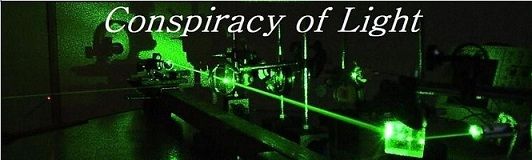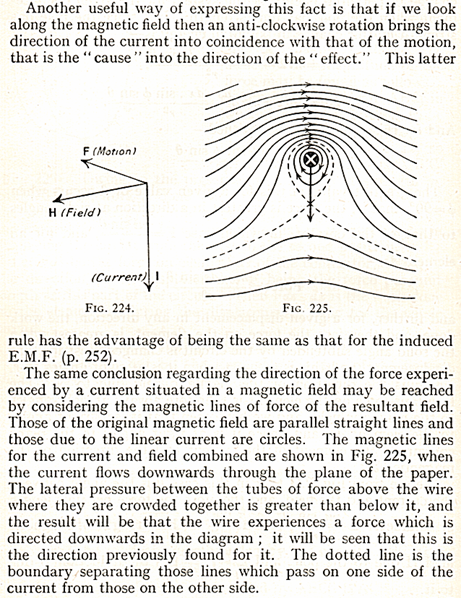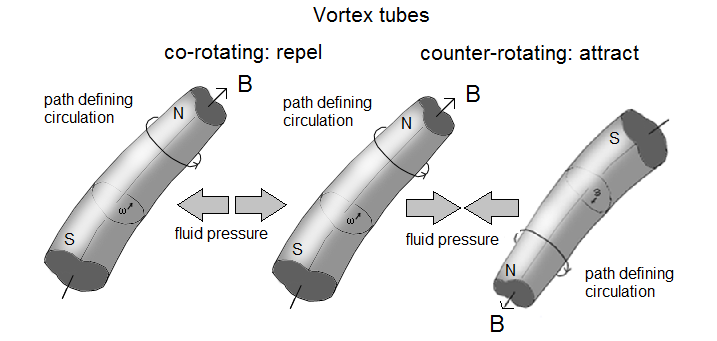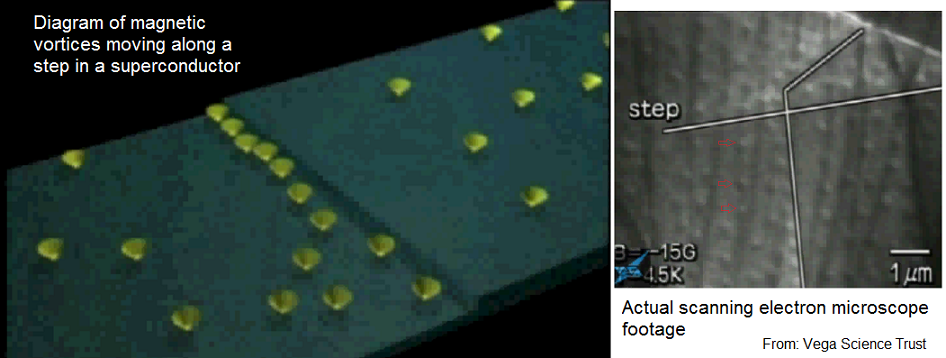The search for  new physics.

Explaining the Lorentz Force Using Magnetic Lines of Force.

Maxwell’s Fluid-Mechanical Approach

D. Marett (2013)

The Lorentz force is usually described abstractly without reference to the physical mechanism by which the force acts in space. Consider for example and diagram of the Lorentz force using the right hand rule, from the website hyperphysics:

1) The right hand rule:A charge moving upwards in the drawing (in the direction of the thumb) experiences a force outward from the palm, when the outstretched fingers point in the direction of the B field.

So what is the actual physical mechanism by which the Lorentz force acts? A physical explanation can be hard to find. We found one in an old physics textbook by S.G. Starling, Electricity and Magnetism for Degree Students (7th Ed., 1941) p. 240, reproduced in Fig. 2:

Fig. 2:In Starlings, explanation, magnetic lines are considered “tubes of force”. He explains that there is a pressure between these tubes of force. Note from the diagram that where the B field of the wire and the external B field point in opposite directions, there is attraction. As Starling says, “an anti-clockwise rotation brings the direction of the current into coincidence with that of the motion, that is the “cause” into the direction of the “effect.” This premise results directly from fluid mechanics. The Lorentz force is derived from James Clerk Maxwell’s equations, and Maxwell’s physical model of Faraday’s lines of force were of vortices in a fluid medium. They obey Helmholtz’s theorems of vortex motion. So ultimately, the Maxwellian physical model of magnetic lines of force is a fluid dynamic model. Quoting James Clerk Maxwell from “On Physical Lines of Force”, P. 455:

“Let us now suppose that the phenomenon of magnetism depend on the existence of a tension in the direction of the lines of force, combined with a hydrostatic pressure; in other words, a pressure greater in the equatorial than in the axial direction: the next question is, what mechanical explanation can we give of this inequality of pressure in a fluid or mobile medium? The explanation which most readily occurs to the mind is that the excess of pressure in the equatorial direction arises from the centrifugal force of vortices or eddies in the medium having their axes in the directions parallel to the lines of force. “

As is known from vortex theory, counter-rotating vortices attract, and co-rotating vortices repel. Quoting from Wikipedia:

“Parallel magnetic lines of force traveling in the same direction repel one another. Parallel magnetic lines of force traveling in opposite directions tend to unite with each other and form into single lines traveling in a direction determined by the magnetic poles creating the lines of force.” This is shown diagrammatically in Fig. 3 below:

Fig. 3:The Lorentz force diagram can now be drawn as shown below in Fig. 4 to illustrate the phenomenon in terms of the force exerted by the magnetic lines.

Fig. 4: The Lorentz force visualized as an interaction between magnetic tubes.The positive charge moving vertically through the magnetic lines of force generates a magnetic field around itself by the right hand rule. The lines of this field are horizontal, in the same place as the field lines of the magnet. The lines of the charge and the lines of the magnet are co-rotating on the far side, and thus repel one another (leading to a pressure on the far side). The lines on the near side are counter-rotating, and thus attract one another. As a consequence, the particle experiences a force to the near side, with the dark arrow shown.

Magnetic Tubes of Force – real or Imaginary?

With the advent of Einstein’s treatment of electrodynamics, the magnetic tube of force has been relegated to an imaginary entity. There may be some good reasons to question this modern approach.  A must see video on the subject is Akira Tonamura’s “Electron Waves Unveil the Microcosmos.”(Vega Science Trust).   In the video Dr. Tonamura succeeds in visualizing bunches of magnetic lines of force

Fig.5: The late Akira Tonamura showing a model of a magnetic line bunch (Vega Science Trust).trapped in a superconductor, a model of which is shown in Fig. 5. The magnetic lines of force behave as if they are real structures, moving like discrete particles, bumping into obstacles and getting stuck behind walls, etc. This is shown vividly in the video, and example of which is shown below in Fig. 6:

Fig. 6: Magnetic lines of force moving as discrete units along a step in a superconductor.Further, Akira Tonamura succeeded in visualizing the annihilation of counter-rotating magnetic vortices. Vortices of one orientation were first set up in the superconductor by applying a strong magnetic field. Once established, the field was then turned off, and some vortices persisted on the surface (often bunched up in cues, waiting to reach the edge of the superconductor). Then the opposite magnetic field was applied, generating the reverse vortices on the same slab of material. When these counter-rotating vortices encounter each other, they annihilate, as shown below in Fig. 7. The video shows actual video footage form the electron microscope of the process in action.

Fig. 7: Counter-rotating magnetic vortices annihilating on a superconductor surface.Another issue arises with the relativistic approach to magnetic fields. The problem is related to co-moving particles. If magnetic field lines were real, then co-moving particles should generate magnetic fields around themselves which interact if they are close enough to each other, via the Lorentz force. This would be because the particles would form magnetic fields relative to the medium of space (after Maxwell). However, as Einstein would explain it, magnetic fields are only visible between particles if there is a velocity difference between them – co-moving particles should experience no Lorentz force between them, since the Lorentz force would be a function of their velocity difference only. However, the phenomenon of Z-pinch does arise in co-moving particles, attributed to the Lorentz force, meaning that co-moving particles can see and react to each others magnetic fields, even when there should be no velocity difference. This could be evidence that magnetic field lines are not relative phenomenon after all.

****************